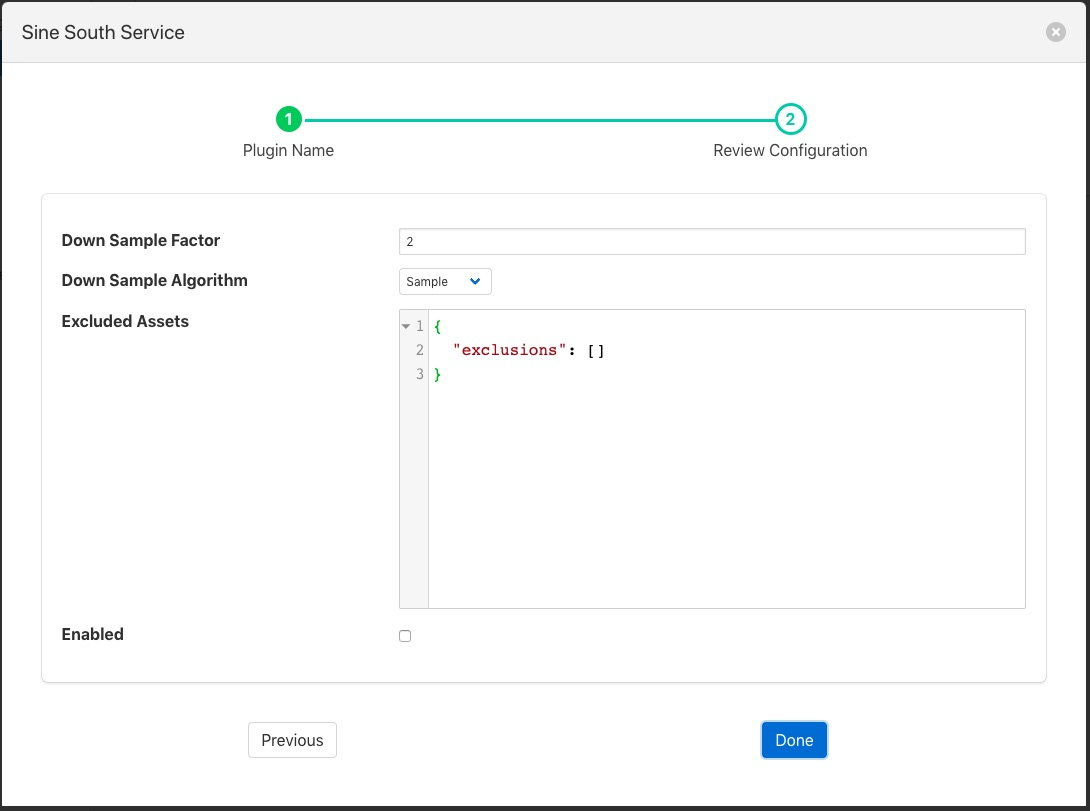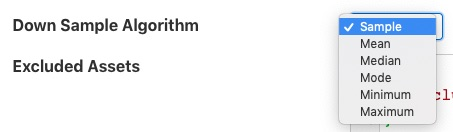# Down Sample Filter¶

The foglamp-filter-downsample filter is a mechanism to reduce the amount of data ingested, it allows the effective data rate to be reduced by a given factor, for example to have the data rate you select a down sample factor of 2, to get a third the rate you select a down sample factor of 3. There are a number of algorithms available to select the value to be sent.

• Sample - the first value in the sample is used as the value for the sample set.

• Mean - the average value in the down sampled set is sent as the down sampled value.

• Median - the mathematical median value is sent as the down sampled value. This is the number found by sorting the sample and choosing the mid point of the sample.

• Mode - the mathematical mode value is sent as the down sampled value. This is the number that appears most often in the sample.

• Minimum - the minimum value in the sample is sent forward.

• Maximum - the maximum value in the sample is sued as the sample value.

Downsample filters are added in the same way as any other filters.

• Select the downsample plugin from the list of available plugins.

• Click Next and you will be presented with the following configuration page